Algebra 1 : Statistics and Probability

Example Questions

← Previous 1 3 4 5 6 7 8 9 70 71

Example Question #1 : Statistics And Probability

What is the mean of 44, 22, 134, and 200?

144

100

88

66

100

Explanation:

To find the mean, you must add all of the numbers together and divide by the amount of numbers. In this case there are four numbers so, we must deivide the total sum by 4.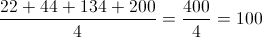Example Question #2 : Statistics And Probability

Calculate the mean of the following numbers: 11, 13, 16, 13, 14, 19, 13, 13Explanation:

First, calculate the sum of all of the numbers.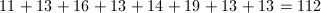Next, divide by the total number.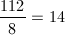Example Question #3 : Statistics And Probability

Find the mean of this number set: 2, 5, 6, 7, 7, 3Explanation:

The numbers add up to 30. To find the mean, divide by the number of numbers (6) and you get a mean of 5. Mean is a fancy way of saying average.

Example Question #4 : Statistics And Probability

The class average in a class of 15 is 86%. If one additional student earns a 100% in the class, what is the new class average.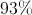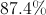There is not enough information to answer this question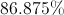Explanation:

We can treat this as if the entire class had exactly 86% as their average, so the new average is: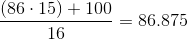Example Question #5 : Statistics And Probability

What is the mean of the following numbers?

88,99,31,47,68,27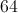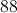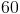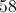Explanation:

To find the mean you add all of the numbers together and divide it by the amount of numbers. In this case there are six numbers so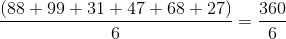The answer is.

Example Question #6 : Statistics And Probability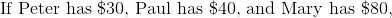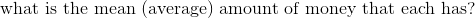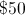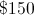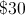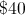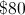Explanation: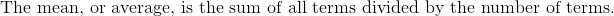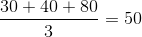Example Question #7 : Statistics And Probability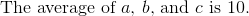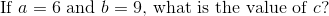Explanation: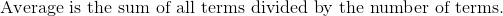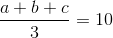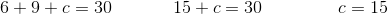Example Question #8 : Statistics And Probability

The sum of the elements of a data set is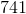; the mean of the elements is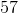. How many elements are there in the set?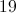It is impossible to tell from the imformation given.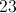Explanation:

The mean,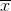, of a data set is the sum of the elements,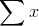,divided by the number of elements,.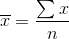We know that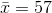and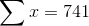. Now we can solve for.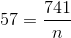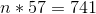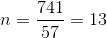Example Question #9 : Statistics And Probability

Two standard dice are rollled. What is the probability that the sum of both dice is greater than?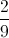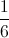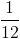Explanation:

There aretotal possible outcomes (sides on one die timessides on the other). The following are the possible outcomes that are greater than.andand5 andandandandThere arepossible outcomes greater than, out ofpossible outcomes total; thus, there is a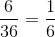chance of rolling greater than.

Example Question #10 : Statistics And Probability

Is the following method for collecting data biased? If so, why?

A company would like to assess the approval of their new product. To determine if customers were happy with their product, they placed a link on their website to all consumers to opt-in to provide their opinion.

Yes; this is an example of exclusion bias.

Yes; this is an example of selection bias.

Yes; this is an example of analytical bias.

No; the collection method is not biased.